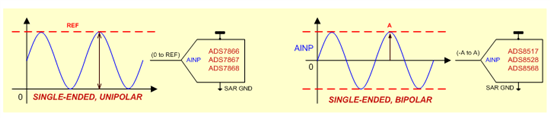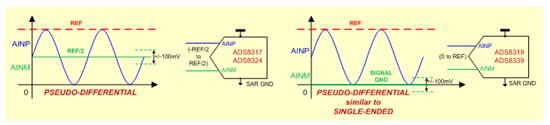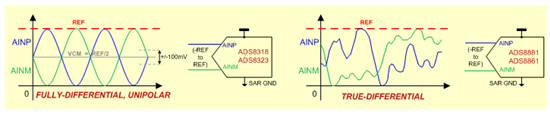This technical article was updated on July 23, 2020.

Did you know that the input signal might affect how you select the best successive approximation register (SAR) analog-to-digital converter (ADC) for your application?

When we hear the word, “input,” there are several things that immediately jump into our heads – like frequency, amplitude, sinusoidal, sawtooth, etc. – all of which are relevant questions to ask when optimizing the signal conditioning.

However, one thing that many do not consider up front is the actual input type of the SAR ADC.  In this blog, I will focus on the three types of SAR inputs:  single-ended, pseudo-differential, and differential inputs, and how you can employ these in your application. In future blogs, I’ll discuss performance differences and some of the key practical considerations that must be kept in mind to obtain the optimal input performance.

Single-ended input is the most simple of the three input types, because the ADC has only one input. As long as the signal fed is within the range specified on input pin, the SAR digitizes the input with respect to SAR ground (see Figure 1).Figure 1:  Single-Ended Conversion Example

While the majority of single-ended SAR ADCs can handle unipolar signals, some are designed to handle bipolar signals with amplitude (A) that can easily exceed the power supply. Some support one channel, while others can support multiple channels. One common application that uses a single-ended ADC input is supply voltage monitoring.

Here’s some additional information on the single-ended input SAR ADCs used in Figure 1:

 Part number Resolution Sample rate ADS8568 16 bit 500 kSPS ADS8517 16 bit 200 kSPS ADS8528 12 bit 650 kSPS ADS7866 12 bit 200 kSPS ADS7867 10 bit 280 kSPS ADS7868 8 bit 280 kSPS

Pseudo-differential SAR ADCs have two input pins; however, it is called “pseudo-differential” because a proper ADC conversion happens when one input remains at a fixed DC voltage (typically REF/2) while the other input can accept a dynamically changing input signal. The difference signal between the two inputs (AINP-AINM) is then converted into a digital code. Typically, a head room of +/-100mV is provided for variations in inputs. Figure 2 illustrates this and a unique case in which the fixed input (AINM) is tied to signal ground, making it similar to a single-ended input type.Figure 2:  Pseudo-Differential Input Configuration

One of the most common applications that employs this configuration is shunt monitoring, where the voltage on one side of a series resistor is measured with respect to a fixed DC voltage and converted back into a current.

Examples of pseudo-differential input SAR ADCs, as used in Figure 2:

 Part number Resolution Sample rate ADS8319 16 bit 500 kSPS ADS8317 16 bit 250 kSPS ADS8339 16 bit 250 kSPS ADS8324 14 bit 50 kSPS

Fully differential input SAR ADCs accept two inputs, where one input is complementary to the other (see Figure 3).  The differential signal between the two inputs (VDIFF = AINP – AINM) is converted.

In most differential input SARs, there is restriction on the common mode voltage (VCM = (AINP + AINM)/2) for the ADC inputs, which translates to a fixed DC offset for both signals (typically REF/2 with tolerance of +/-100mV).

However, as is shown in Figure 3, there are newer SAR ADCs with a unique input stage that can handle common mode voltages that can vary from 0 to REF. Such an input is referred to as true-differential input.Figure 3:  Fully Differential and True Differential Input Configuration

Fully differential SAR ADCs support bipolar inputs and/or multiple channels, similar to single-ended SAR ADCs. Applications that use transformer output employ fully differential input SARs.

Here’s more background on the fully differential input SAR ADCs used in Figure 3:

 Part number Resolution Sample rate ADS8881 18 bit 1 MSPS ADS8861 16 bit 1000 kSPS ADS8318 16 bit 500 kSPS ADS8323 16 bit 500 kSPS

Now that I’ve explained some of the most common SAR ADC input types, be sure to read my two-part series on how performance varies between these input types

• Hello, what will happen if I connect a full differetial signal to pseudo-differential ADC or if I connect a pseudo differential signal to full differential ADC

• very good!

• Great question e-bird! It depends.

1. Typically, for fully differential input SARs, the common mode voltage Vcm = (AINP+AINM)/2 is fixed. There will be a row in the data sheet that shows the range for Vcm. You cannot connect signal to one input and ground the other (unless it is stated explicitly in the PDS) as the comon mode voltage will keep varying with the input.

2. For true-differential input SARs, you can connect single ended signals directly as the common mode voltage can vary (has to be be with in the spec).  ADS8861/ADS8881 are some of the SARs that have true-differential inputs. By doing so a portion of the dynamic range will be lost.

3. In some SARs such as ADS8321/ADS8323, the inputs can be driven by single-ended signals or differential signals. This will be stated explicitly in the datasheet. Please note that in such devices, the REF voltage will be half of analog supply voltage (AVdd) and hence the overall dynamic range will be half of what can be achieved with fully differential devices where REF can go upto AVdd.

Hope that helps!

• Nice article! For a fully differential input SAR ADC which requires complementary input, if I connect a single ended signal directly, will the SAR ADC still give the correct code with limited dynamic range, or the ADC won’t work at all with wrong code?

• dispositivos fundamentales para el desarrollo electrónico